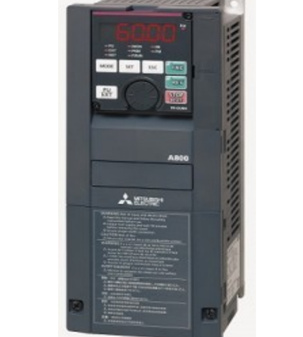# How to set parameters for Mitsubishi 820 & 840 VFD?The 800 series Mitsubishi drives are high performance drives it’s a 480volt drive and if we look at the above image we can see a keypad in this VFD and it is the part which we use to set the parameters. The drive can be set in net mode, external mode and in parameter mode, in external mode the drive will be controlled with analog signals and dry contacts, the net mode is for the communications. In order to set the parameter we must set the VFD mode into parameter unit mode so that we can control this VFD, so push the PU external in the drive, we can see the switches for the parameter unit mode. After this we must push mode till a “P” appears in the display which is a programming parameter select the parameter 1 and push set. The parameter 1 is to set the maximum frequency so set the maximum frequency that is required, we can change the frequency by turning the small wheel that we can see in this VFD. The maximum frequency range is sixty

Then we can go to the parameter 2 which is to set the minimum frequency and then set the minimum frequency that is required and press set after changing the frequency then it will be set. Parameter 3 is the frequency and set it according to the required application. Then go to parameter 7 to set the acceleration time and set it according for the application, we can vary the values or select the values by turning the small wheel that can be seen in the above image.

Then press set in the drive thus the acceleration parameter is set then press again the set then it will go to the deceleration time which is parameter 8, then set the deceleration value that is required for the application. Then we can go to the parameter 9 and it is the motor full load amps and we should set this value by checking the motor name plate, after that we should go to the parameter 19 which is the base frequency voltage, which is the motor’s voltage and it must also be set by checking the motor name plate. Then we should go to parameter 14 which can be used to set the constant torque mode it can also be set to variable. Then by using the mode in the VFD set the speed and press set after setting the speed then press run the motor will start and it will run according to the speed that we set.